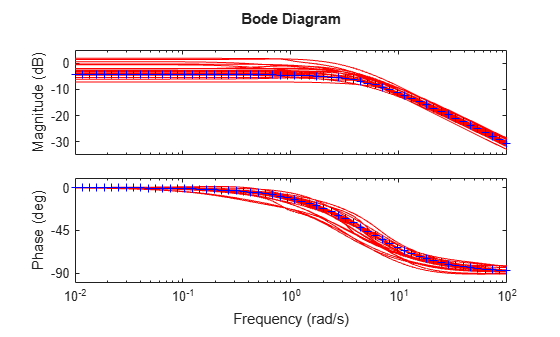# ufrd

Uncertain frequency response data model

## Syntax

```ufrd_sys = ufrd(M,freqs) ufrd_sys = ufrd(M,freqs,frequnits) ufrd_sys = ufrd(M,freqs,frequnits,timeunits) ```

## Description

Uncertain frequency response data models (`ufrd`) arise when combining numeric `frd` models with uncertain models such as `ureal`, `ultidyn`, `umargin`, or `uss`. A `ufrd` model keeps track of how the uncertain elements affect the frequency response. Use `ufrd` for robust stability and worst-case performance analysis.

There are three ways to construct a `ufrd` model:

1. Combine numeric `frd` models with uncertain models using model arithmetic. For example:

```sys = frd(rand(100,1),logspace(-2,2,100)); k = ureal('k',1); D = ultidyn('Delta',[1 1]); ufrd_sys = k*sys*(1+0.1*D)```

`ufrd_sys` is a `ufrd` model with uncertain elements `k` and `D`.

2. `ufrd_sys = ufrd(M,freqs)` converts the dynamic system model or static model `M` to `ufrd`. If `M` contains Control Design Blocks that do not represent uncertainty, these blocks are replaced by their current value. (To preserve both tunable and uncertain Control Design Blocks, use `genfrd` instead.)

Use `ufrd_sys = ufrd(M,freqs,frequnits)` to specify the frequency units of the frequencies in `freqs`. The argument `frequnits` can take the following values:

• `'rad/TimeUnit'`

• `'cycles/TimeUnit'`

• `'rad/s'`

• `'Hz'`

• `'kHz'`

• `'MHz'`

• `'GHz'`

• `'rpm'`

Use `ufrd_sys = ufrd(M,freqs,frequnits,timeunits)` to specify the time unit of `ufrd_sys` when `M` is a static model. `timeunits` can take the following values:

• `'nanoseconds'`

• `'microseconds'`

• `'milliseconds'`

• `'seconds'`

• `'minutes'`

• `'hours'`

• `'days'`

• `'weeks'`

• `'months'`

• `'years'`

3. Use `frd` to construct a `ufrd` model from an uncertain matrix (`umat`) representing uncertain frequency response data. For example:

```a = ureal('delta',1,'percent',50); freq = logspace(-2,2,100); RespData = rand(1,1,100) * a; usys = frd(RespData,freq,0.1)```

## Examples

collapse all

Compute the uncertain frequency response of an uncertain system with both parametric uncertainty (`ureal`) and uncertain gain and phase (`umargin`). Create the uncertain frequency response by building a `uss` model using uncertain dynamics, and then extracting its response at a specified set of frequencies.

```p1 = ureal('p1',5,'Range',[2 6]); p2 = ureal('p2',3,'Plusminus',0.4); F = umargin('F',1.2); A = [-p1 0;p2 -p1]; B = [0;p2]; C = [1 1]; usys = uss(A,B,C,0)*F; freqs = logspace(-2,2,60); usysfrd = ufrd(usys,freqs); ```

Plot random samples and the nominal value of the uncertain frequency response.

```rng(0); % for reproducibility bode(usysfrd,'r',usysfrd.NominalValue,'b+') ```### Topics

Introduced before R2006a

## SupportGet trial now